# F.2 Electron-Phonon Interaction

The coupling of electrons and nuclei in the lattice to the external sources is given by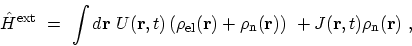(F.24)

where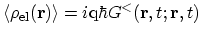is the density of electrons. The density of the nuclei in the lattice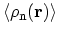is represented as a sum of local charge densities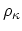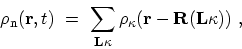(F.25)

where the actual positions of the nuclei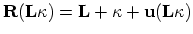, is defined in terms of the equilibrium lattice vector, the basis vector within the unit cell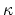, and the lattice displacement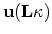. In case of bare nuclei,would approximately be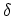functions. However, it is more convenient to consider rigid ion cores instead of bare nuclei. In this casedenotes the charge density of the ion cores.

For a simple derivation of the electron-phonon interaction, one has to add an additional external source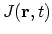in (F.24) , which couples to the charge density of the nuclei and is merely a mathematical trick, see (F.32)-(F.35). With similar steps for deriving (F.7), one can show that under the HAMILTONian in (F.24) the effective potential can be written as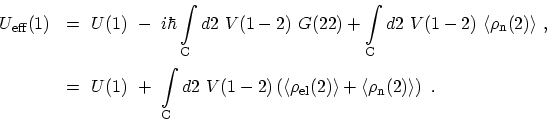(F.26)

The aim is the calculation of the total linear response of the system, including the contribution from the nuclei, i.e. the variation of the total electrostatic potential with the external potential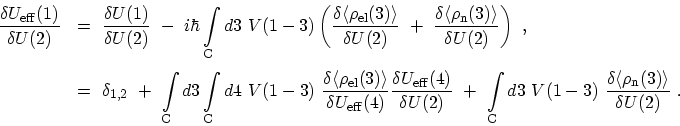(F.27)

Solving with respect to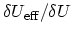, one obtains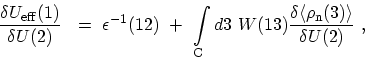(F.28)

where the dielectric function is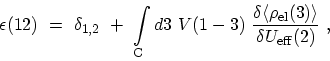(F.29)

and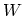is the screened interaction. The derivative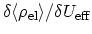differs from the purely electronic polarization which is introduced in Appendix F.1.1, owing to the phonon contribution to the total potential. Neglecting this phonon contribution to the polarization function is one of the ingredients of the adiabatic approximation . This approximation reduces the dielectric function and the screened interaction in (F.28) to the purely electronic quantities which are introduced in (F.20) and (F.21). The next step is the calculation of the lattice contribution. Similar to the GREEN's function for electrons, one can consider the expectation value of the density of nuclei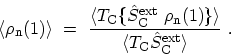(F.30)

With similar steps for deriving (F.5), the density response of the nuclei under the action of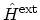can be calculated as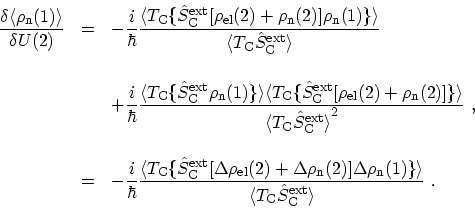(F.31)

In the last step, the deviation operator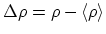is introduced. Furthermore, the relation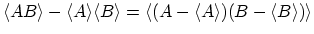is used. Now the additional external field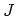comes into play, which allows us to eliminate the mixed electron-nuclei contribution. By steps completely analogous to those used before, one finds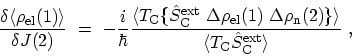(F.32)

which together with (F.31), yields the result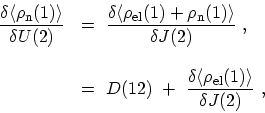(F.33)

where the density-density correlation function of the nuclei is defined as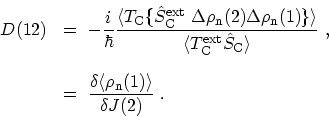(F.34)

One can again apply the chain rule to (F.33) to eliminate the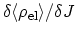contribution.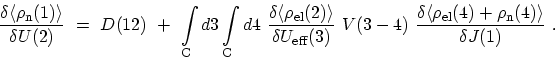(F.35)

Making use of the relation (F.33) once more, one can solve the resulting equation with respect to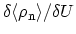and express the solution in terms of the dielectric function. After insertion in (F.28), this yields the total dielectric screening function as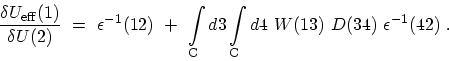(F.36)

The desired effective electron-electron interaction induced by lattice vibrations is thus finally given by(F.37)

Therefore, the problem of electron-phonon interaction is reduced to the replacement of the electronically screened interaction introduced in Appendix F.1.1 by the effective interaction (F.37).

Subsections

M. Pourfath: Numerical Study of Quantum Transport in Carbon Nanotube-Based Transistors